parallelogram wkst
Last Name *
Period *
Find measure of angle Q *
1 point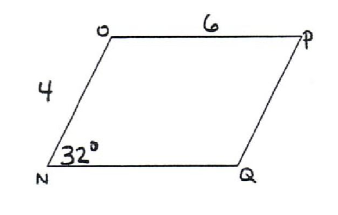Find measure of angle p *
1 pointFind measure of angle 4
1 point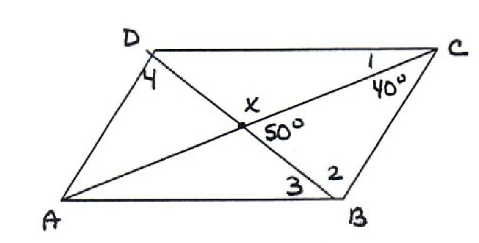Find x *
1 point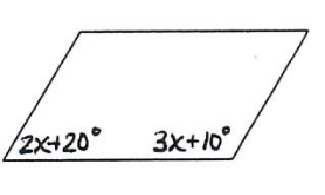x = *
1 point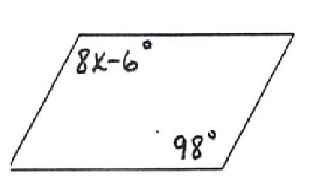*
1 point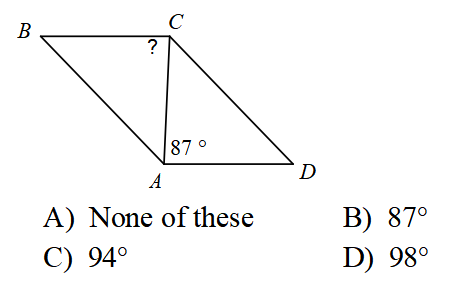*
1 point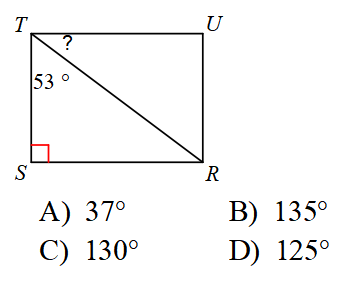*
1 point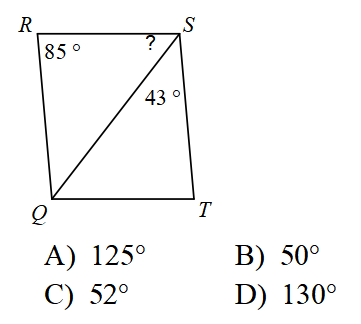*
1 point*
1 point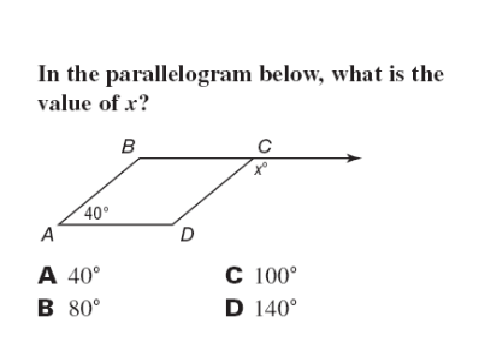Find length of segment BE *
1 point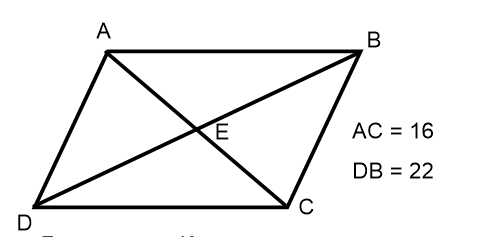Find length of segment AC. In this parallelogram the diagonals are congruent
1 point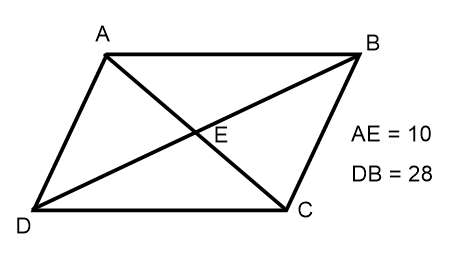Find the SUM of angles z and y *
1 point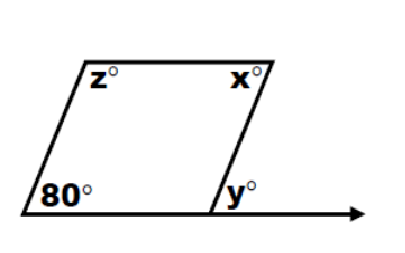Find the measure of angle z *
1 point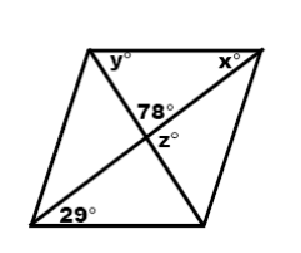Find the length of side AC
1 point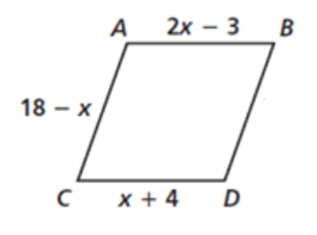Never submit passwords through Google Forms.
This content is neither created nor endorsed by Google. Report Abuse - Terms of Service - Privacy Policy